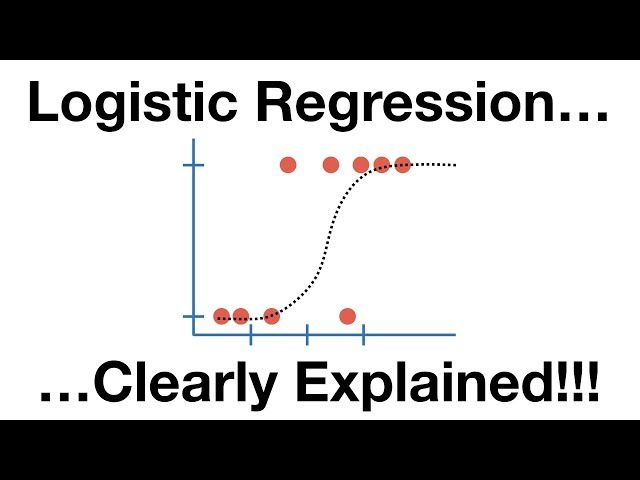# What is Logistic regression

##### Logistic Regression: Understanding the Concept and its Applications in Machine Learning

Introduction

Logistic regression is one of the most popular techniques used in machine learning for classification problems. It is a statistical method used to analyze and model the relationship between a binary dependent variable and one or more independent variables. The key concept behind logistic regression is the logistic function which helps to predict the probability of an event occurring or not occurring.

Understanding Logistic Function

The logistic function is also known as the sigmoid function. It is an S-shaped curve that maps any input value to a value between 0 and 1. The formula for the logistic function is given by:

$$f(x) = \frac{1}{1 + e^{-x}}$$

This function is used to model the probability of a binary dependent variable. It takes the independent variables as inputs and returns the predicted probability of the dependent variable being 1 (true). If the predicted probability is greater than 0.5, the event is predicted to occur, otherwise, it is predicted not to occur.

Logistic Regression Model

The logistic regression model is used to model the relationship between the independent variables and the binary dependent variable. It is a type of regression analysis used to solve classification problems.

The logistic regression model assumes that there is a linear relationship between the independent variables and the log odds of the dependent variable. The log odds are defined as:

$$log\frac{P(y=1)}{1-P(y=1)}$$

Where P(y=1) is the probability of the dependent variable being 1 (true). The logistic regression model uses this relationship to estimate the coefficients of the independent variables that maximize the likelihood of the observed data.

##### Applications of Logistic Regression

Logistic regression is widely used in various fields for classification problems. Some of the common applications are:

• Medical Diagnosis: Logistic regression is used to diagnose diseases based on symptoms and patient data.
• Credit Scoring: It is used by banks and financial institutions to predict the probability of default by a borrower.
• Marketing: Logistic regression is used for customer segmentation and targeting based on their response to marketing campaigns.
• Fraud Detection: It is used to detect fraudulent transactions in the financial industry.
• Sports Analytics: Logistic regression is used to predict the outcome of sports events based on team performance and other factors.

• Logistic regression is easy to implement and interpret.
• It can handle both categorical and continuous independent variables.
• It provides a good balance between bias and variance.
• It can handle nonlinear relationships between the independent and dependent variables.

• Logistic regression assumes linearity between the independent variables and the log odds of the dependent variable.
• It may not work well with highly correlated independent variables.
• Logistic regression may not be the best model for complex classification problems with multiple dependent variables.
• The accuracy of the logistic regression model depends on the quality of the training data.
##### Conclusion

Logistic regression is a powerful technique used in machine learning for classification problems. It is widely used in various fields such as medical diagnosis, credit scoring, marketing, fraud detection, and sports analytics. Logistic regression provides a good balance between bias and variance and is easy to interpret. However, it may not work well with highly correlated independent variables and may not be the best model for complex classification problems with multiple dependent variables. Therefore, it is important to carefully evaluate the requirements of the problem before choosing a machine learning algorithm.